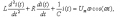# Guys, need help with matlab: can't plot graph (differential equations)

#### Gudsaf

Joined May 23, 2013
1
So, good day.
I had problem with matlab: can't plot graph.
Need to say u, i from Russia, maybe my language bad, so sorry))
Maybe u can give advice - where (what forum) can help me?

My equation:My code:
Rich (BB code):
T = [0 5e-6];
i0 = [0 0];
[t, I] = ode45('qweq',T,i0);
% печатаем график i(t) / print i(t)
figure
plot(t,I(:,1))
grid on
legend('i(t)')
xlabel('t')
ylabel('i')
title('График зависимости тока от времени')
% печатаем график di/dt / print di/dt
figure
plot(t,I(:,2))
grid on
legend('di/dt')
xlabel('dt')
ylabel('di')
title('График зависимости di по dt')
Function:
Rich (BB code):
function F = qweq(t,I)
R0 = 2;
L = 1e-6;
C = 0.001e-6;
f = 1e6;
k = 8e10;
Um = 1;

w = 2*pi*f;
R = R0*(1-k*I(1)^2);

F = zeros(2,1);
F(1) = I(2);
F(2) = ( Um*w*cos(w*t) - (1/C)*I(1) - R*I(2) )/L;
if i set k as 0, all ok. But in task k must be 8e10...